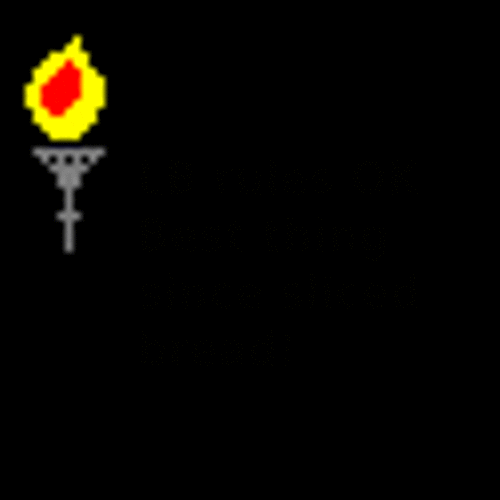# Bresenham's algorithm to draw straight lines.When a computer video system is required to draw a line, it has to 'light up' the appropriate pixels. However these lie on a 2D matrix of positions, so while horizontal and vertical may be easily drawn, at other angles it has to step along and/or up to give the best representation.

Liberty BASIC is pretty good at calling underlying Windows routines, so as users we do not see this going on- a line will magically appear using the fastest method and best approximation.

Horizontal or vertical- easy!-But at angles we have best to take a pattern of alongs and ups.It may help to use fractional tones so the eye is better fooled into seeing a smooth line- anti-aliasing. This leads rto Bresenham's method.

## Particular relevance to Liberty BASIC

LB has a set of turtle commands, which make simple drawing very easy. However there are a number of drawbacks for accurate representation and convenience. There is no access to the current ( x, y) of the drawing pen, and its position is stored rounded off to an integer, so rounding errors add up. Thus doing 20 'go's of 1 behaves as expected, but not 20 'go's of 0.3 or 1.3. And the geometric figures like squares or stars may not exactly join, so if you apply a fill it leaks out. And while you can alter a line width or color as a whole, you can't change it along a line- say fading the color or narrowing the width, or creating dot/dash effects.

The introductory animation shows that by implementing your own Bresenham routine you can examine each pixel which the algorithm wishes to draw and inhibit it for a chosen background color. So an invisible logo, written in a near-black colour, is slowly revealed...

## LB implementation

```    nomainwin

WindowWidth  =530
WindowHeight =550

graphicbox #w.gb, 10, 10, 500, 500

global hdc

open "Bresenham" for graphics_nsb as #w

#w "trapclose quit"

h = hwnd( #w.gb)
calldll #user32, "GetDC", h as ulong, hdc as ulong

#w.gb "goto 100 270 ; down ; fill 1 0 0 ; color 0 0 0 ; backcolor 1 0 0"
#w.gb "font Times_Roman bold italic 90": #w.gb "\  LB" +chr\$( 13) +"rules!"
#w.gb "color 255 255 0 ; rule "; _R2_COPYPEN

for jf =1 to 2000
x1 =-100 +int( 700 *rnd( 1))
y1 =   0 +int( 500 *rnd( 1))
x2 =-100 +int( 700 *rnd( 1))
y2 =   0 +int( 500 *rnd( 1))
call bresenham x1, y1, x2, y2
next jf

#w.gb "getbmp scr 0 0 500 500"
'bmpsave "scr", "bresenhamInverse.bmp"

wait

sub quit h\$
close #h\$
calldll #user32, "ReleaseDC", hw as ulong, hdc as ulong   'release the DC
end
end sub

sub bresenham x1, y1, x2, y2    '    Inputs are x1, y1, x2, y2: destroys value of x1, y1
dx  = abs( x2 - x1): sx = -1: if x1 < x2 then sx = 1
dy  = abs( y2 - y1): sy = -1: if y1 < y2 then sy = 1

er  = 0 -dy: if dx > dy then er = dx
er  = int( er / 2)

[more]  g   = getPixel( x1, y1)     '   can only write on longcolor 1 or own colour...
'if ( g = 1) or ( g = bg) then #w.gb "set "; x1; " "; y1
'if y1 >250 then #w.gb  "color red" else #w.gb "color blue"
p       =int( ( y1 +100) /600 *255)
c\$      =str\$( p) +" " +str\$( int( ( x1 +100) /700 *255)) +" " +str\$( 255 -p)
#w.gb  "color " +c\$
if ( g <> 1)               then #w.gb "set "; x1; " "; y1

scan

if ( ( x1 = x2) and ( y1 = y2))                              then exit sub
'if ( ( x2 > 490) or ( x2 < 10) or ( y2 > 490) or ( y2 < 10)) then exit sub    '   should not happen!
e2  = er

if ( e2 > 0 -dx) then er = ( er - dy): x1 = ( x1 + sx)
if ( e2 <    dy) then er = ( er + dx): y1 = ( y1 + sy)
goto [more]

end sub

function getPixel( x, y)
calldll #gdi32, "GetPixel", hdc as ulong, x as long, y as long, pixcol as ulong
getPixel =pixcol
end function

'function MakeRGB\$( cc)
'    blu         =int(  cc /256 /256)
'    grn         =int( ( cc -blu *256 *256) / 256)
'    red         =int( cc -blu *256 *256 -grn *256)
'    MakeRGB\$    =str\$( red) +" " +str\$( grn) +" " +str\$( blu)
'end function

```

```
```